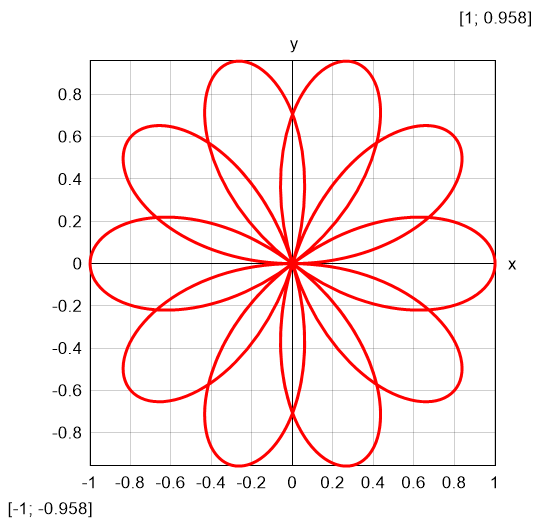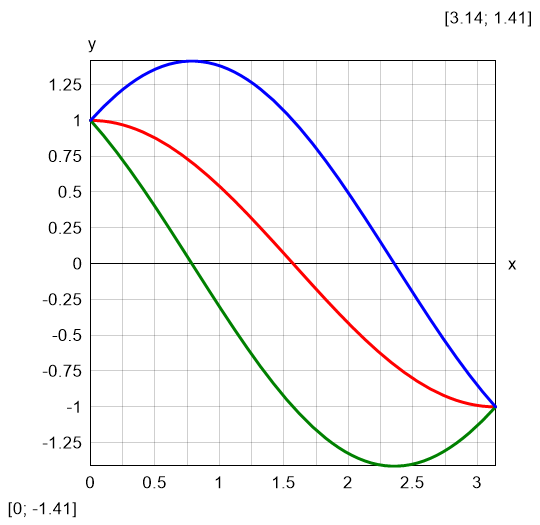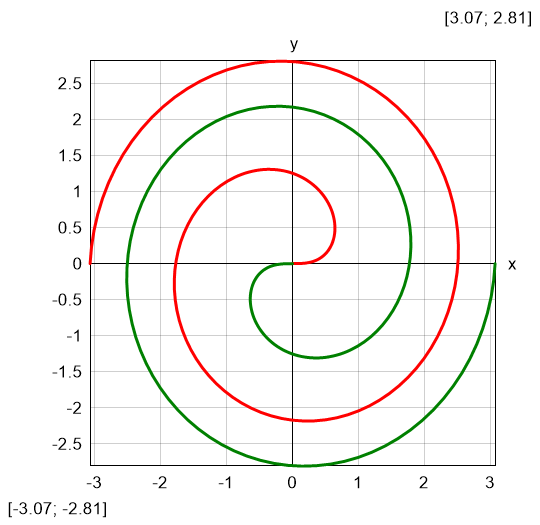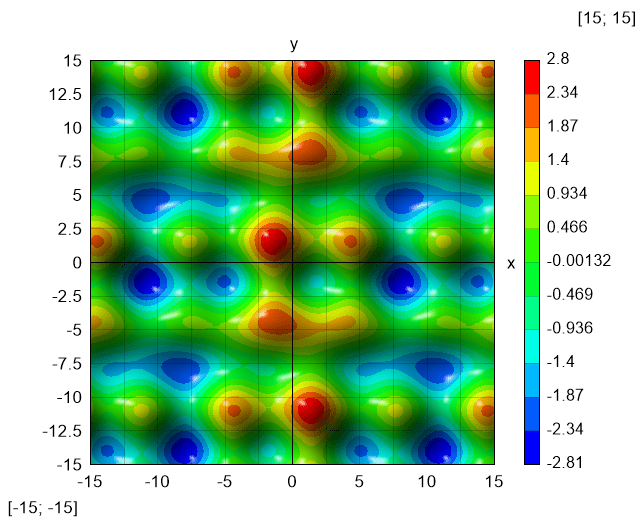Topic: Expressions

## Plotting

Besides functions, Calcpad provides special commands for advanced operations. They accept functions and expressions as arguments and perform plotting, iterative solutions and numerical methods. Their names start with "\$" to be distinguished from normal functions. Their parameters must be enclosed in curly brackets: "{" and "}". Such is the plotting command \$Plot. It can plot a function of one variable in the specified interval. It has the following format:

`\$Plot{y(x) @ x = a : b}`

, where:

• y(x) - the function to be plotted. Instead of y(x) you can put any valid expression. It will be used to calculate the ordinate values;
• x - the name of the variable along the abscissa. You can put here only a single name. It is not required to define the variable preliminary;
• a and b are the limits of the interval for the x variable. Instead of a and b you can put numbers, variables or any valid expressions.

For example, if you enter: `\$Plot{x^2 - 5*x + 3 @ x = -1:6}`, you will get the following result:The above command plots only one function of one variable at a time. There are also other formats that you can use:

`\$Plot{x(t)|y(t) @ t = a : b}` - parametric plot: both coordinates are functions of a parameter;
`\$Plot{y1(x) & y2(x) & ... @ x = a : b}` - multiple: plots several functions on a single graph;
`\$Plot{x1(t)|y1(t) & x2(t)|y2(t) & ... @ t = a : b}` - multiple parametric;
`\$Map{f(x; y) @ x = a : b & y = c : d}` - draws a 2D color map of a 3D surface, defined by f(x; y).

The \$Plot{...} function must be the first thing in a line. You can have only spaces and tabs beofre, not even comments. Otherwise, the program will return an error. Any text after the closing bracket "}" will be ignored. Plotting supports only real numbers. You can use it in complex mode, only if x and y are real and the function returns real result along the plotting interval.

You can specify the size of the plot area by defining two variables: PlotWidth and PlotHeight in pixels. The default values are PlotWidth = 400 and PlotHeight = 250.

The \$Map{...} function can work with different color palettes. Select the preferred one from the "Palette" combo box on the bottom of the program window. If you select the "Smooth" checkbox, the scale will be displayed as a smooth gradient. Otherwise, the program will draw color strips. You can also add 3D effects to the graph by selecting the "Shadows" checkbox. You can also specify light direction by the respective combo.

Examples of different plotting methods are provided bellow:

 Parametric Equation: `r(θ) = cos(5/2*θ)` `\$Plot{r(θ)*cos(θ)|r(θ)*sin(θ) @ θ = 0:6*π}` Multiple Equation: `y1(θ) = cos(θ) - sin(θ)` `y2(θ) = cos(θ) + sin(θ)` `\$Plot{cos(θ) & y1(θ) & y2(θ) @ θ = 0:π}` Result: "rose" curveResult: leaf by three trigonometric functionsMultiple parametric Equation: `x(θ) = sqr(θ)*cos(θ)` `y(θ) = sqr(θ)*sin(θ)` `\$Plot{x(θ)|y(θ) & -x(θ)|-y(θ) @ θ = 0:3*π}` Color map Equation: `f(x; y) = cos(x/3) + sin(y) - sin(x)*cos(y/4)` `\$Map{f(x; y) @ x = -15 : 15 & y = -15 : 15}` Result: double Fermat spiralResult: 2D waves